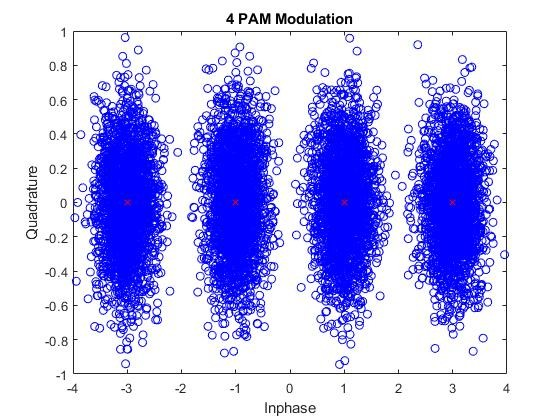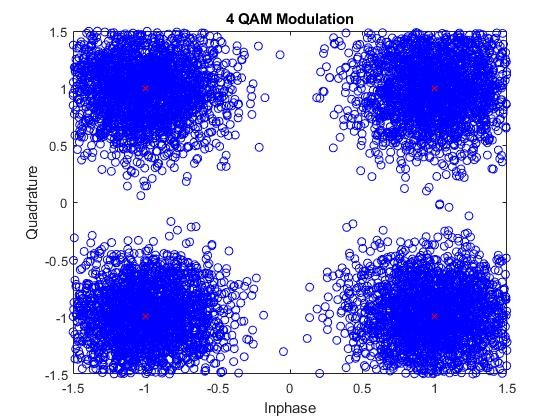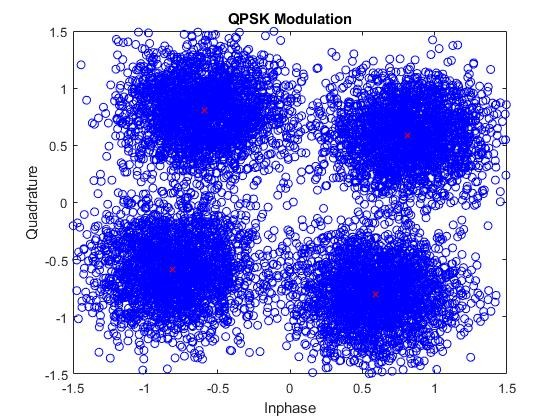In this post, we turn our attention to the robustness of a modulation technique in the presence of noise. (Chapter 4.2.4 and 4.2.5, SDR 4 ENGINEERS (TRAVIS F.))

Code explanation: Noise introduced by a transmission medium can potentially result in symbols being decoded in error. In the following MATLAB script, we will examine the behavior of how the introduction of noise can obfuscate the true identity of an intercepted symbol. Specifically, we will compare the originally transmitted symbols and the noisy received symbols using a visual representation referred to as a signal constellation diagram, which plots the locations of symbols across a 2-axis plot with an in-phase axis and a quadrature axis. Notice that we are considering three different waveforms in this example: 4- PAM, 4-QAM, and QPSK. For each of these waveforms, we generated an alphabet of different symbols that each can produce. The randomly generated binary data streams representing in-phase and quadrature information are mapped to these different waveform symbols for each modulation scheme. Then, we introduce Gaussian noise to the three transmissions using the randn function.

The output: